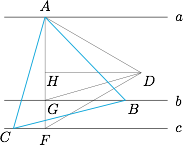# 每日一题作图与论证作法
(1) 作$AF\perp c$且分别交直线$b,c$于点$G,F$；
(2) 以$AF$为底边作正三角形$ADF$；
(3) 过$D$作$AF$的垂线，垂足为$H$；
(4) 将射线$AD$按$\angle GDH$旋转交直线$b$于点$B$；
(5) 以$AB$为底边作正三角形$ABC$．